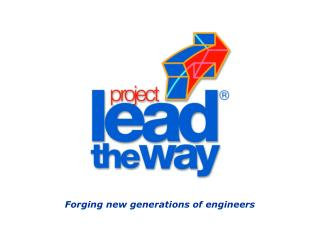DownloadDownload PresentationForging new generations of engineers

# Forging new generations of engineers

Download Presentation## Forging new generations of engineers

- - - - - - - - - - - - - - - - - - - - - - - - - - - E N D - - - - - - - - - - - - - - - - - - - - - - - - - - -
##### Presentation Transcript

1. Forging new generations of engineers

2. The English System of Measurement

3. How precise do I need to be? • We need to be able to accurately measure to the .001th .125

4. .125 = 1/8” .250 = 1/4” .375 = 3/8” .500 = 1/2” .625 = 5/8” .750 = 3/4” .875 = 7/8” 1. = 1” .0625 = 1/16” .1875 = 3/16” .3125 = 5/16” .4375 = 7/16” .5625 = 9/16” .6875 = 11/16” .8125 = 13/16” .9375 = 15/16” Decimal to Fraction Conversions

5. Tools used in Precision Measurement • Gage Blocks: These are used throughout industry as primary standards and have accuracy to a few millionths of an inch.

6. Gage Blocks

7. Micrometers Sleeve Ratchet Stop Thimble

8. Dial Caliper

9. Dial Indicators